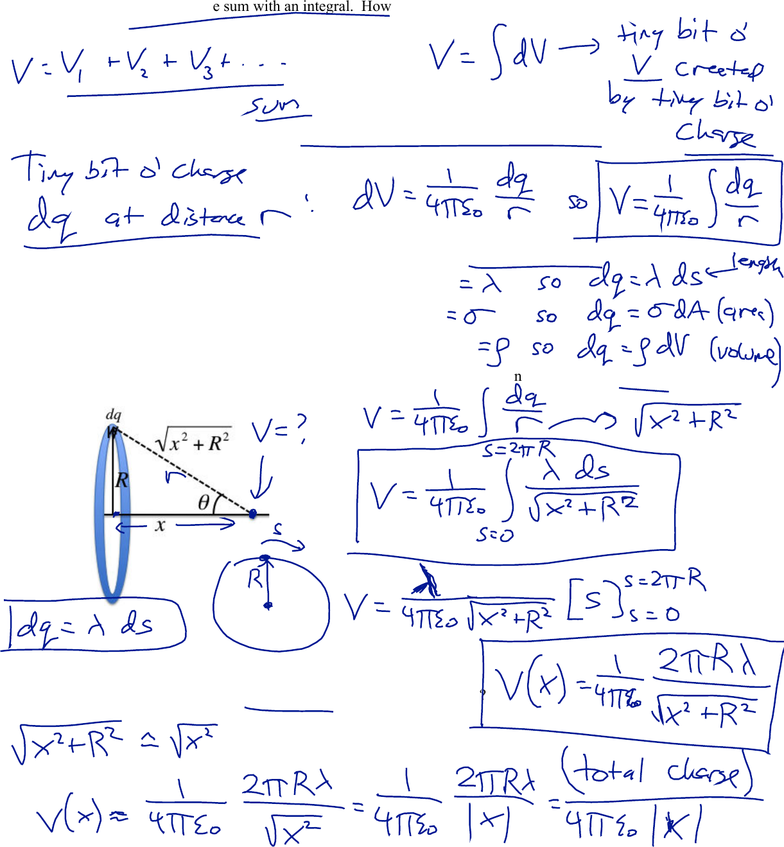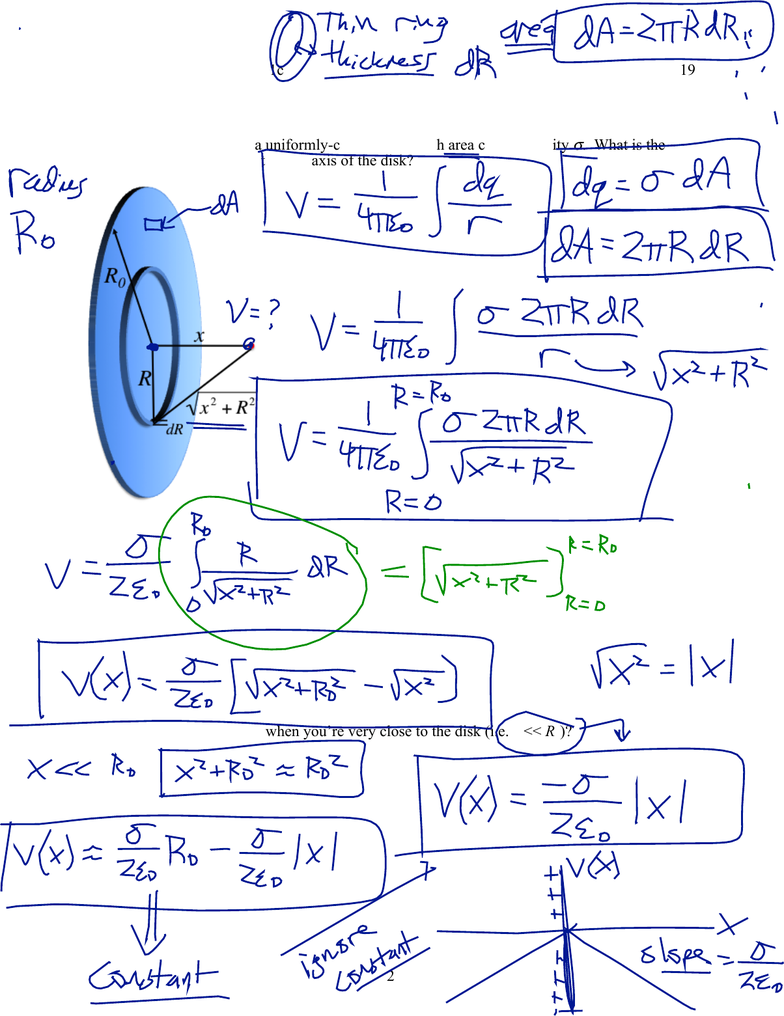Study Guides (400,000)
US (230,000)
Harvard (700)
(20)
Final

# Physical Sciences 3 Lecture 3: Lecture 1cExam

Department
Physical Sciences
Course Code
Physical Sciences 3
Professor
Roxanne Guenette
Study Guide
Final

This preview shows pages 1-3. to view the full 10 pages of the document.Physical Sciences 3: Lecture 1c
February 5, 2019
1
Current and Resistance
An insulator has essentially no charge that is “free to move.” Although a potential
gradient will exert a force on charges in an insulator, the charges remain bound and do
not flow. A conductor contains charge that is free to move. If there is a potential
gradient in a conductor, these charges will feel a force and will flow in response.
A conductor at equilibrium will have the same potential everywhere. An empty cavity
inside a conductor at equilibrium will also have the same uniform potential. Thus, there
will be no potential gradient inside a conductor at equilibrium.
If a potential difference is applied across a conductor, charge will flow. The flow of
charge is called current. The current i that results can be given by any of the following
relationships:
From resistance (Ohm’s Law):
From resistivity
ρ
or conductivity
σ
: or
For ions in solution, the total conductivity is the sum of the conductivity due to each
species of ion in the solution. (The solution must overall be electrically neutral.) The
conductivity of a particular species of ion is modeled simply as:
: c is the concentration, q is the charge, and f is the drag coefficient of the ion
Learning objectives: After this lecture, you will be able to…
1. Describe the difference between a conductor and an insulator.
2. Explain why any conductor at equilibrium will have the same electric potential
everywhere on its surface and inside it.
3. Derive a simple model to show how the electric current carried by ions in a solution is
related to:
• the charge of the ion, q
• the drag coefficient of the ion, f (this is viscous drag like Stokes’s Law)
• the concentration of ions, c
• the potential gradient across the solution, ΔV/L
• the cross-sectional area of the solution, A
4. Describe the behavior of conductors in terms of conductivity or resistivity.
5. Derive Ohm’s Law that relates current, voltage, and resistance
i=ΔV
R
i=ΔV
ρ
A
L
i=ΔV
σ
A
L
σ
=cq2
f

Only pages 1-3 are available for preview. Some parts have been intentionally blurred.Physical Sciences 3
February 5, 2019
Activity 1: Continuous Charge Distributions: Ring of Charge
Often, we will want to model a continuous distribution of charge. In this case, we’ll need
to replace the sum with an integral. How can we express the superposition principle?
So what will be the tiny (infinitesimal) potential created by a tiny bit of charge?
Usually, we’ll express the tiny bit of charge in terms of a density of charge:
For a linear density (charge per unit length):
For an area density (charge per unit area):
For a volume density (charge per unit volume):
What the potential on the axis of a thin ring of charge, with linear charge density
λ
?
What if you’re very far away from the ring (i.e. x >> R)?

Only pages 1-3 are available for preview. Some parts have been intentionally blurred.Physical Sciences 3: Lecture 1c
February 5, 2019
2
Continuous Charge Distributions: Disk of Charge
Now let’s consider a uniformly-charged disk with area charge density
σ
. What is the
potential at any point on the axis of the disk?
What about the limit when you’re very close to the disk (i.e. x << R0)?